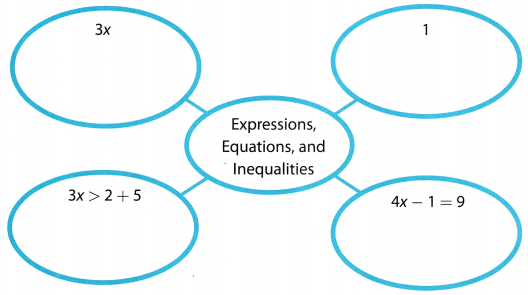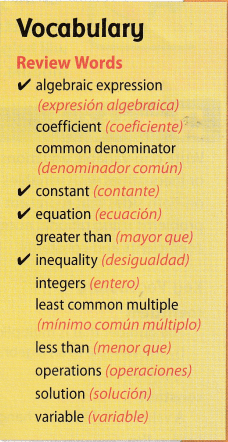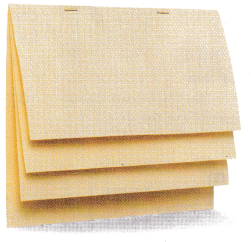# Texas Go Math Grade 8 Module 11 Answer Key Equations and Inequalities with the Variable on Both Sides

Refer to our Texas Go Math Grade 8 Answer Key Pdf to score good marks in the exams. Test yourself by practicing the problems from Texas Go Math Grade 8 Module 11 Answer Key Equations and Inequalities with the Variable on Both Sides.

## Texas Go Math Grade 8 Module 11 Answer Key Equations and Inequalities with the Variable on Both Sides

Find the LCD.

Question 1.
8, 12 ________
(List the multiples of each number)8 : 8, 16, 24, 32, …..
(Choose the least multiple the lists have in common)12 : 12, 24, 36, 48. …
LCD(8, 12) = 24

Question 2.
9, 12 ________
List the multiples of each number
9 : 9, 18, 27, 36, …….
12 : 12, 24, 36, 48, 56, …
Choose the least multiple the lists have in common
LCD : 36

Question 3.
15, 20 _______
List the multiples of each number
15 : 15, 30, 45, 60,…
20 : 20, 40, 60, 80,…
Choose the least multiple the lists have in common
LCD : 60

Question 4.
8, 10 ________
List the multiples of each number
8: 8, 16, 24, 32, 40, 48,…
10: 10, 20, 30, 40, 50,…
Choose the least multiple the lists have in common
LCD : 40

Find the product.

Question 5.
0.683 × 100 ________
Count the zeros in 100 : 2 zeros
0.683 × 100
Move the decimal point 2 places to the right
68.3

Question 6.
9.15 × 1,000 ________
Count the zeros in 1000 : 3 zeros
9.15 × 1000
Move the decimal point 3 places to the right
9150

Question 7.
0.005 × 100 ________
Count the zeros in 100 : 2 zeros
0.005 × 100
Move the decimal point 2 places to the right
0.5

Question 8.
1,000 × 1,000 ________
Count the zeros in 1000 : 3 zeros
1000 × 1000
Move the decimal point 3 places to the right
1,000,000

Write an algebraic equation for the sentence.

Question 9.
The difference between three times a number and 7 is 14. __________
Given,
The difference between three times a number and 7 is 14
Represent the unknown with a variable Times means multiplication
The difference between three times x and 7 is 14
Difference means subtraction
3x – 7 is 14
Place the equal sign
3x – 7 = 14

Question 10.
The quotient of five times a number and 7 is no more than 10. __________
The quotient of five times x and 7 is no more then 10.
Represent the unknown with a variable.
(5x) ÷ 7 ≤ 10 (Times means multiplication.)

Question 11.
14 less than 3 times a number is 5 more than half of the number. ___________
Given
14 less than 3 times a number is 5 more than half of the number.
Represent the unknown with a variable Times means multiplication
14 less than 3 times x is 5 more than $$\frac{1}{2}$$x
less than means subtraction
3x – 14 is 5 more than $$\frac{1}{2}$$x
3x – 14 is 5 + $$\frac{1}{2}$$x
Place the equal sign
3x – 14 = 5 + $$\frac{1}{2}$$x

Visualize Vocabulary

Use the ✓ words to complete the bubble map. You may put more than one word in each oval.Understand Vocabulary

Complete the sentences using the review words.

Question 1.
A value of the variable that makes an equation true is a ___________.
A value of the variable that makes an equation true is a “solution“.

Question 2.
The set of all whole numbers and their opposites are ____________.